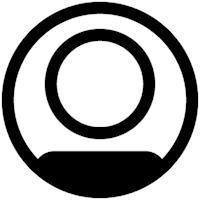# SPSS Statistics

View Only

## Syntax for new variable where the value of variables 1 and 2 are within 3 units from variable 3#### Lars OlsenWed April 14, 2021 12:10 AM#### Rick MarcantonioWed April 14, 2021 08:46 AM• #### 1.  Syntax for new variable where the value of variables 1 and 2 are within 3 units from variable 3

Posted Wed April 14, 2021 12:10 AM
Hello

I have three scale variables with values ranging from 1-10 (whole numbers only).

I would like to create a new variable based on the following conditions:

1. The value of variable 1 should be 6 or higher,
2. The value of variable 2 and variable3  should be maximum three numbers higher than or lower than the value of variable 1.

Any help would be greatly appreciated!

Lars Olsen

------------------------------
Lars Olsen
------------------------------

• #### 2.  RE: Syntax for new variable where the value of variables 1 and 2 are within 3 units from variable 3

Posted Wed April 14, 2021 08:46 AM
If I understood correctly, I think this should do it.

`data list free /v1 to v3.`
`begin data.`
`1 10 5`
`6 5 8`
`7 8 5`
`6 2 6`
`6 3 9`
`end data.`

`compute v4= (v1 ge 6) & (abs(v2-v1) le 3) & (abs(v3-v1) le 3).`

`list.`

------------------------------
Rick Marcantonio
Quality Assurance
IBM
------------------------------

• #### 3.  RE: Syntax for new variable where the value of variables 1 and 2 are within 3 units from variable 3

Posted Wed April 14, 2021 11:36 AM
Thank you very much for the suggestion, and please accept my apologies for not being clear enough in the original post!

What I want to achieve is the following.

The values in the new variable, var4, should be either 0 or 1 based  on the following conditions:

1. The value of var1 (scale 1 to 10)  should be equal to or greater than 6
2. The value of var2 (scale 1 to 10 ) should either be equal to or 1,2 or 3 numbers less than or greater than the value of var1
3. The value of var3 (scale 1 to 10 ) should either be equal to or 1,2 or 3 numbers less  han or greater than the value of var1

All cases that meet alle three conditions gets value 1, else value 0 in the new var4.

All the best,
Lars

------------------------------
Lars Olsen
------------------------------